## 3.3.1 Buffon's Needle Experiment

A famous "needle-throwing" experiment first proposed by Buffon in 1777 provides a good example of probabilistic modeling from a geometrical point of view [BUFF 771. Suppose we have a large flat surface that has been ruled with a series of equidistant parallel lines separated by a distance d. (For instance, one might imagine this to be the alternating red and white striped field of the American flag.) An experimenter throws a needle of length l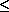d d "at random" onto the surface and we wish to compute the probability that the needle intersects one of the parallel lines (e.g., lies on both red and white stripes of the flag). We suppose that the surface is large enough so that the needle always lands on it and that boundary effects are negligible.

To model this experiment, just as with other probabilistic experiments, there are four things we must do:

STEP 1: Define the random variables of interest.

STEP 2: Identify the joint sample space.

STEP 3: Determine the joint probability distribution over the sample space.

STEP 4: Work within the sample space to determine the answers to any questions about the experiment.

1. Random variables. Any experimental outcome is fully described by the position of the needle on the surface. A convenient depiction of this position is given by two random variables (see Figure 3.17):

Y = distance from the center of the needle to the closest of the equidistant parallel lines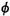= angle of the needle, measured with respect to the parallel lines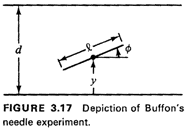With the position random variables defined in this way, we clearly have 0Yd/2 and 0. This choice of random variables is motivated in part by symmetries that can be exploited and in part by the simple probability laws that govern their behavior. Other sets of random variables could be selected, some that would lead to tractable analyses and some that would not. For instance, we could define Y to be the distance from the center of the needle to the next "southern" parallel line (assuming that the lines run east-west). In this case 0Yd, and the analysis follows very closely the analysis given below. However, we could also define the position of the needle by the (x,y) coordinates of its two end points; this would lead to an unnecessarily complicated analysis. In analyzing an experiment, the efficient selection of a reasonable set of random variables often comes only with modeling experience.

2. Joint sample space. For the position random variables we have selected, the joint (Y,) sample space corresponds to the rectangle 0yd/2, 0(Figure 3.18).3. Joint probability distribution. To determine the joint probability density function fY,(y,) we must interpret the words "at random," describing the throwing mechanism. This is often not an easy thing to do, as the example in the next section will illustrate. In the absence of other information, it is plausible to assume here that the angular position of the needle is uniformly distributed between 0 and, and thus the marginal pdf for the angle is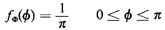Similarly, it is reasonable to assume that the location of the center of the needle is uniformly distributed, implying that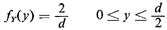To obtain the joint pdf, fy,(· , ·)it would be "nice" ifand Y were independent, for then the joint pdf would simply be the product of the two marginals. Indeed, in this case, there is no reason to assume that knowing the needle's angle would affect one's knowledge about its center position or conversely, and thus the independence assumption can be invoked. Thus,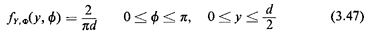4. Working in the joint sample space. We are asked for the probability that the needle intersects one of the parallel lines. To answer this question, all we need do is identify the set of points in the joint (Y,) sample space that corresponds to "intersection of a parallel line" and integrate the joint pdf over that set of points to obtain the desired probability. To do this, we must define "intersection of a parallel line" in terms of the random variables in the sample space. By examining Figure 3.17, it is clear that intersection will occur if "y is sufficiently small for a given angle." Assuming the situation shown in Figure 3.17, the coordinate of the lower end of the needle is y - (1/2) sin. If this coordinate is negative, intersection will occur. Thus, intersection occurs for all points in the (Y,) sample space satisfying the inequality y(l/2) sin. Integrating over this set (see Figure 3.19), we obtain the desired probability:A somewhat remarkable property of this result is that it contains a fundamental constant of nature,. If one threw needles many times (and independently) at a lined surface, one could estimate. Exercise 3.4-. Buffon's Needle, Another Way Redo this analysis assuming that the random variable Y is the distance from the center of the needle to the next "southern" parallel line (so that 0Yd). Exercise 3.5: Buffon's Longer Needle Solve the Buffon needle problem for the case in which the needle is unrestricted in length, (This requires an analysis of the case I > d.)